SSC  >  Logical Reasoning - Test 7

# Logical Reasoning - Test 7

Test Description

## 25 Questions MCQ Test SSC CGL Tier 1 Mock Test Series | Logical Reasoning - Test 7

Logical Reasoning - Test 7 for SSC 2022 is part of SSC CGL Tier 1 Mock Test Series preparation. The Logical Reasoning - Test 7 questions and answers have been prepared according to the SSC exam syllabus.The Logical Reasoning - Test 7 MCQs are made for SSC 2022 Exam. Find important definitions, questions, notes, meanings, examples, exercises, MCQs and online tests for Logical Reasoning - Test 7 below.
Solutions of Logical Reasoning - Test 7 questions in English are available as part of our SSC CGL Tier 1 Mock Test Series for SSC & Logical Reasoning - Test 7 solutions in Hindi for SSC CGL Tier 1 Mock Test Series course. Download more important topics, notes, lectures and mock test series for SSC Exam by signing up for free. Attempt Logical Reasoning - Test 7 | 25 questions in 15 minutes | Mock test for SSC preparation | Free important questions MCQ to study SSC CGL Tier 1 Mock Test Series for SSC Exam | Download free PDF with solutions
 1 Crore+ students have signed up on EduRev. Have you?
Logical Reasoning - Test 7 - Question 1

### Select the related word from the given alternatives. moq : lnpr ∷ ceg : ?

Detailed Solution for Logical Reasoning - Test 7 - Question 1

→ Both moq and lnpr, together make a small part of English alphabetical series.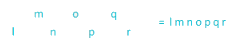→ Likewise ceg and bdfh, together make a small part of English alphabetical series.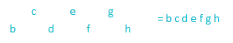Logical Reasoning - Test 7 - Question 2

### Select the related word from the given alternatives. Mahatama Gandhi  :1948 ∷ Nelson Mandela : ?

Detailed Solution for Logical Reasoning - Test 7 - Question 2

Mahatama Gandhi died on 30 January 1948. Similarly Nelson Mandela died on
05 December 2013.
Hence, “2013” is correct option.

Logical Reasoning - Test 7 - Question 3

### Select the related numbers from the given alternatives. 34 : 59 :: 57 : ?

Detailed Solution for Logical Reasoning - Test 7 - Question 3

The logic here is as follows,
⇒ 34 + 32 + 42 = 34 + 9 + 16 = 59
Similarly,
⇒ 57 + 52 + 72 = 57 + 25 + 49 = 131
Hence, "131" is correct option.

Logical Reasoning - Test 7 - Question 4

Select the odd word from the given alternatives.

Detailed Solution for Logical Reasoning - Test 7 - Question 4

→ Except Potato and Raddish, all other pairs are useful to make drinking related Recipes.

Logical Reasoning - Test 7 - Question 5

Select the odd word from the given alternatives.

Detailed Solution for Logical Reasoning - Test 7 - Question 5

There are live word present in OliveLivewire and Livewire but there is no live in Living.
Therefore Alone is odd word among them.

Logical Reasoning - Test 7 - Question 6

Select the odd figure from the given alternatives.

Detailed Solution for Logical Reasoning - Test 7 - Question 6

In image of option 1)
⇒ (4 × 5) + (5 × 3) – (4 × 3)
⇒ 20 + 15 – 12
⇒ 23
In image of option 2) ​
⇒ (7 × 6) + (6 × 2) – (2 × 7)
⇒ 42 + 12 – 14
⇒ 40 but in diagram it is given as 27.
In image of option 3) ​
⇒ (9 × 6) + (6 × 8) – (8 × 9)
⇒ 54 + 48 – 72
⇒ 30
In image of option 4) ​
⇒ (3 × 2) + (2 × 1) – (1 × 3)
⇒ 6 + 2 – 3
⇒ 5
Therefore image of option 2) is odd among them.

Logical Reasoning - Test 7 - Question 7

Which of the following group of numbers follows the trend?

111O, O1111, 11111O, __________

Detailed Solution for Logical Reasoning - Test 7 - Question 7

→ ‘O’ comes at start and last position alternatively and always number of 1’s is increased by one.

Logical Reasoning - Test 7 - Question 8

Which of the following words follow the trend of the given word list?

Oasis, Noise, Drone, Allot, _______

Detailed Solution for Logical Reasoning - Test 7 - Question 8

In given series “O” is moving in next position with each increasing term.
Oasis, Noise, Drone, Allot,
So now with next term “O” will move to 5th position.
Now in option only Audio has “O” as it fifth letter.

Logical Reasoning - Test 7 - Question 9

Find the missing term in diagram shown below?
CK   16    9     JR

OS   24    19    TX

KM   ?     ?     PV

Detailed Solution for Logical Reasoning - Test 7 - Question 9

Putting A = 1, B = 2, C = 3, ...., M = 13, ....., X = 24, Y = 25, Z = 26, we have:
In First row,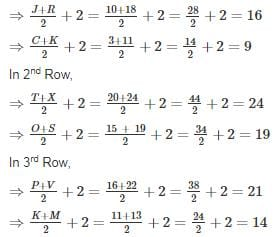Hence, 21, 14 is missing term.

Logical Reasoning - Test 7 - Question 10

In a certain code, ‘256’ means ‘red colour chalk’; ‘589’ means ‘green colour flower’ and ‘245’ means ‘White colour chalk’. Which digit in that code means ‘white’?

Detailed Solution for Logical Reasoning - Test 7 - Question 10

Based on given information we can summarise information in below diagram,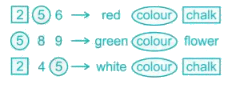Hence, Code for ‘White’ will be ‘4’

Logical Reasoning - Test 7 - Question 11

In the question below are given three statements followed by two conclusions numbered I and II. You have to take the given statements to be true even if they seem to be at variance from commonly known facts. Read both the conclusions and then decide which of the given conclusions logically follows from the given statements disregarding commonly known facts.

Statements:
Some walls are huts
Some huts are houses
All houses are roofs

Conclusions:
I. Some houses are walls
II. No roof is a hut

Detailed Solution for Logical Reasoning - Test 7 - Question 11

If we analyze the statements given in the question, we get the following: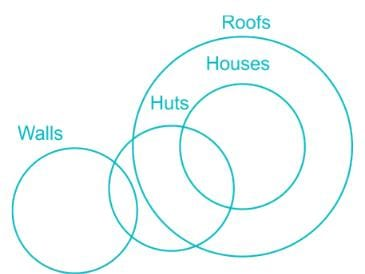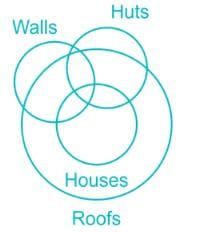From the above analysis:
Conclusion I is not always true.
Conclusion II is never true.

Logical Reasoning - Test 7 - Question 12

Sunita rode her scooty northwards, then turned left and after taking another left, rode 4 km. She found herself exactly 2 km west of her starting point. How far did she ride northwards initially?

Detailed Solution for Logical Reasoning - Test 7 - Question 12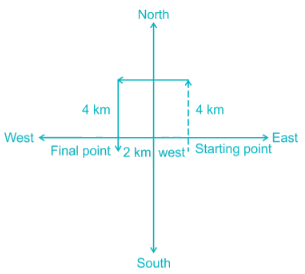Thus Sunita rode 4 km towards north initially.

Logical Reasoning - Test 7 - Question 13

If the letters N, S, C, E, O, U & F are numbered 1, 2, 3, 4, 5, 6 & 7 respectively. Select that combination of numbers so that letters arranged accordingly, form a meaningful word.

Detailed Solution for Logical Reasoning - Test 7 - Question 13

→ Arranging letters according to 3517624,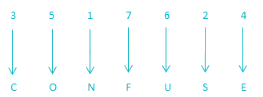→ CONFUSE is a meaningful word which means unable to think clearly.

Logical Reasoning - Test 7 - Question 14

A word is represented by only one set of numbers as gives in any one of the alternatives. The sets of numbers given in the alternatives are represented by two classes of alphabets as shown in the given two matrixes. The columns and rows of Matrix – I are numbered from 0 to 4 and that of Matrix – II are numbered from 5 to 9. A letter from these matrices can be represented first by its row and next by its column, for example ‘Z’ can be represented by 20, 24 etc and ‘Y’ be represented by 55, 85 etc similarly, you have to identify the set for the word ‘SHARP’.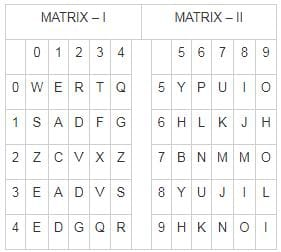Detailed Solution for Logical Reasoning - Test 7 - Question 14

1) 10, 65, 11, 44, 56 ⇒ S, H, A, R, P
2) 65, 21, 33, 69, 34 ⇒ H, C, V, H, S
3) 55, 21, 98, 01, 10 ⇒ Y, C, O, E, S
4) 34, 65, 11, 44, 22 ⇒ S, H, A, R, V
Therefore, “10, 65, 11, 44, 56” are set of number which represent word “SHARP”

Logical Reasoning - Test 7 - Question 15

Six men A, B, C, D, E and F are sitting on a round conference table. B is sitting between E and F and opposite to A. C is sitting to the immediate left of F. Determine who is sitting opposite to F.

Detailed Solution for Logical Reasoning - Test 7 - Question 15

Since six persons are sitting on the round table therefore 6 positions should be fixed on it, as shown in the following diagram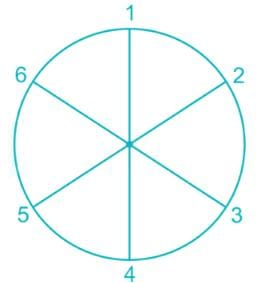Sitting arrangement can be started by placing B between E and F, with F being on his left side, as we need left seat of F to be vacant for C.
Next, A should be placed opposite to B as specified in the question.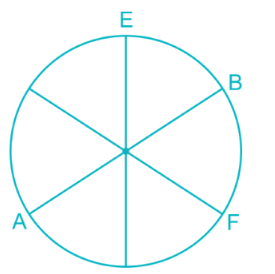Then, C should be placed to immediate left of F.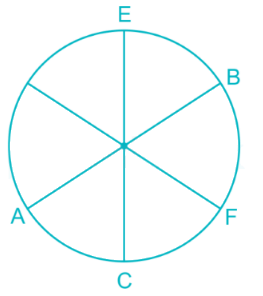It can be observed that only D is left, therefore D can sit on the only available position which is opposite to F. Hence, D is sitting opposite to F.

Logical Reasoning - Test 7 - Question 16

The weights of five boxes are 10, 20, 50, 70, and 90 kilograms. Which of the following cannot be the total weight (in kilograms) of any combination of these boxes?

Detailed Solution for Logical Reasoning - Test 7 - Question 16

→ Taking three boxes weights are 20, 70 and 90 kilograms and the total weight of combination is 180 Kilograms.
→ Taking four boxes weights are 10, 50, 70 and 90 kilograms and the total weight of combination is 220 Kilograms.
→ Taking all boxes weights are 10, 20, 50, 70 and 90 kilograms and the total weight of combination is 240 Kilograms.
→ There is no combination of boxes from which total weight can be 200 kilograms.

Logical Reasoning - Test 7 - Question 17

Insert the correct sign & find out the result:

24 * 7 * 5 * 5 * 8 = 25

Detailed Solution for Logical Reasoning - Test 7 - Question 17

24 * 7 * 5 * 5 * 8 = 25
let’s try all the options:
1) +, ÷, ×, – ⇒ 24 + 7 ÷ 5 × 5 – 8 = 25
⇒ 24 + 7 – 8 = 25
⇒ 23 ≠ 25
2) ÷, +, –, × ⇒ 24 ÷ 7 + 5 – 5 × 8 = 25
⇒ 3.4(approx.) + 5 – 40 = 25
⇒ - 31.6 ≠ 25
3) ×, –, +, ÷ ⇒ 24 × 7 – 5 + 5 ÷ 8 = 25
⇒ 168 – 5 + 5 ÷ 8 = 25
⇒163+58=25⇒163+58=25⇒1304+58=25⇒1304+58=25
⇒ 163.5(approx) ≠25
4) –, ×, ÷, + ⇒ 24 – 7 × 5 ÷ 5 + 8 = 25
⇒ 24 – 7 + 8 = 25
⇒ 25 = 25 ⇒ This is our answer.

Logical Reasoning - Test 7 - Question 18

N is more intelligent than M, M isn’t as intelligent as X. X is more intelligent than Y but not as good as N. Who’s the most intelligent of them all?

Detailed Solution for Logical Reasoning - Test 7 - Question 18

N is more intelligent than M ⇒ N > M
M isn’t as intelligent as X ⇒ X > M
X is more intelligent than Y ⇒ X > Y
X is not as good as N ⇒ N > X
⇒ N > X > Y, M
⇒ N is the most intelligent of them all.

Logical Reasoning - Test 7 - Question 19

Four positions of a dice are given below. Identify which number is on the face opposite 2.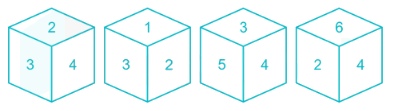Detailed Solution for Logical Reasoning - Test 7 - Question 19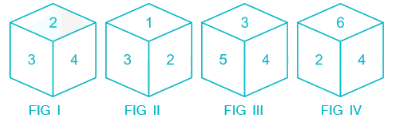Figure I says 3 and 4 cannot be opposite to 2.
Figure II says 1 and 3 cannot be opposite to 2.
Figure IV says 6 and 4 cannot be opposite to 2.
Overall, 1, 3, 4 and 6 cannot be opposite to 2.
So, 5 must be opposite face to 2.

Logical Reasoning - Test 7 - Question 20

From the given answer figures, select the one in which the question figure is hidden/embedded.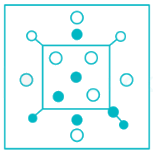Detailed Solution for Logical Reasoning - Test 7 - Question 20

The question figure is hidden in the answer 1).

Logical Reasoning - Test 7 - Question 21

Directions: In question, which answer figure will complete the question figure?Detailed Solution for Logical Reasoning - Test 7 - Question 21Clearly, option1 represents the portion to complete the given question figure.

Logical Reasoning - Test 7 - Question 22

If A @ B means A is father of B, A # B means A is brother of B and A ! B means A is grandson of B, then what does P @ Q # R ! S mean?

Detailed Solution for Logical Reasoning - Test 7 - Question 22

Now draw family tree using following notations: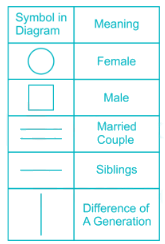→ Breaking the statement P@Q#R!S
P@Q, P is father of Q
Q#R, Q is brother of R
R!S, R is grandson of S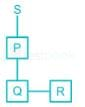P@Q#R!S Means R is son of P and P is son of S.
Therefore, P is son of S is correct option.

Logical Reasoning - Test 7 - Question 23

A piece of paper is folded and punched as shown below in the question figures. From the given answer figures, indicate how it will appear when opened.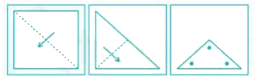Detailed Solution for Logical Reasoning - Test 7 - Question 23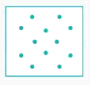Now if we will open paper as it is folded in given question figure then it will appear as it is shown in above question figure.
Hence, image of option 1) is correct answer.

Logical Reasoning - Test 7 - Question 24

In the figure given below, square represents people who are singers, triangle represents actors and circle represents directors. Which set of letters represents people who are both singers and directors?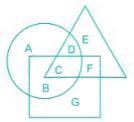Detailed Solution for Logical Reasoning - Test 7 - Question 24

→ People who are both singers and directors, so necessary things is people should both singers and directors either they are actors or not.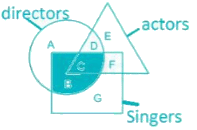Therefore, B and C represent area who are both singers and directors.

Logical Reasoning - Test 7 - Question 25

The writer can get good ideas only when he constantly rejuvenates himself. This rejuvenation comes from new experiences that the writer must take part in.

Q. Which of the following supports the thought?

Detailed Solution for Logical Reasoning - Test 7 - Question 25

Option 1 is the correct answer. The central idea of the sentence is to convey the need for good writers to break monotony and take new experiences, thereby regenerating new ideas. Option 2 is the exact opposite of the idea of taking up new experiences. Option 3 and 4 talk about travelling which is nowhere conveyed in the above sentence.

## SSC CGL Tier 1 Mock Test Series

42 docs|103 tests
 Use Code STAYHOME200 and get INR 200 additional OFF Use Coupon Code
Information about Logical Reasoning - Test 7 Page
In this test you can find the Exam questions for Logical Reasoning - Test 7 solved & explained in the simplest way possible. Besides giving Questions and answers for Logical Reasoning - Test 7, EduRev gives you an ample number of Online tests for practice

## SSC CGL Tier 1 Mock Test Series

42 docs|103 tests